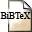# The restricted isometry property of block diagonal matrices for group-sparse signal recovery

## Abstract

Group-sparsity is a common low-complexity signal model with widespread application across various domains of science and engineering. The recovery of such signal ensembles from compressive measurements has been extensively studied in the literature under the assumption that measurement operators are modeled as densely populated random matrices. In this paper, we turn our attention to an acquisition model intended to ease the energy consumption of sensing devices by splitting the measurements up into distinct signal blocks. More precisely, we present uniform guarantees for group-sparse signal recovery in the scenario where a number of sensors obtain independent partial signal observations modeled by block diagonal measurement matrices. We establish a group-sparse variant of the classical restricted isometry property (RIP) for block diagonal sensing matrices acting on group-sparse vectors, and provide conditions under which subgaussian block diagonal random matrices satisfy this group-RIP with high probability. Two different scenarios are considered in particular. In the first scenario, we assume that each sensor is equipped with an independently drawn measurement matrix. We later lift this requirement by considering measurement matrices with constant block diagonal entries. In other words, every sensor is equipped with a copy of the same prototype matrix. The problem of establishing the group-RIP is cast into a form in which one needs to establish the concentration behavior of the suprema of chaos processes which involves estimating Talagrand's γ2 functional. As a side effect of the proof, we present an extension to Maurey's empirical method to provide new bounds on the covering number of sets consisting of finite convex combinations of possibly infinite sets.

## BibTEX Reference Entry```@article{KoBeMa22,
author = {Niklas Koep and Arash Behboodi and Rudolf Mathar},
title = "The restricted isometry property of block diagonal matrices for group-sparse signal recovery",
pages = "333-367",
journal = "Applied and Computational Harmonic Analysis",
volume = "60",
doi = 10.1016/j.acha.2022.03.007,
year = 2022,
}
```Download bibtex-file